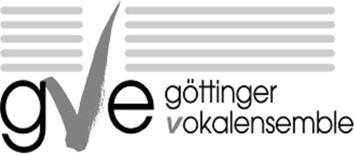# Prof. Dr. Ralf Meyer

My main area of research is noncommutative geometry, a generalisation of our idea of what space and geometry are. This theory is inspired by quantum mechanics. The Heisenberg commutation relation predicts that the position and momentum observables in quantum mechanics form a noncommutative algebra. This noncommutative algebra describes the phase space of a quantum mechanical system. Thus some noncommutative algebras seem to describe geometric objects. Which geometric ideas carry over to such spaces, and how?

Algebraic topology provides many tools to distinguish different spaces by some homological invariants. Most of these invariants do not carry over to noncommutative spaces. The main invariant in that setting is K-theory, which for ordinary spaces classifies the vector bundles over a space. In noncommutative geometry, K-theory is part of a bivariant theory, which provides powerful tools for computation of K-theory and a rich categorical structure. One important aspect of my work is extra structure in bivariant K-theory and how to use it, among others, for the classification of C*-algebras.

Another important homological class of invariant for noncommutative algebras are cyclic cohomology theories. Periodic cyclic cohomology generalises the de Rham homology of smooth manifolds, but only makes sense for algebras that behave like smooth functions. Variants of it like analytic and local cyclic cohomology also give good results for larger algebras like C*-algebras, which behave more like continuous functions. The original periodic cyclic cohomology is computable through its relationship to Hochschild cohomology. One important aspect of my work has been to extend various tools of homological algebra to algebras that carry some functional analytic structure, such as algebras of smooth or holomorphic functions and their noncommutative analogues. I found that bornologies are better behaved than topologies when functional analysis meets algebra. In particular, we may want to compare the homological algebra for two different algebras that describe the same noncommutative space with more or less extra structure. One may be an algebra of polynomial functions, the other an algebra of smooth functions on that noncommutative space. We would expect the homological algebra for these to be related, and my notion of an isocohomological embedding gives one way to make precise how.

Ordinary symmetries of spaces are usually described through group actions. Local symmetries may lead to groupoid actions. In noncommutative geometry, however, the more general notion of space should also lead to drastically different types of geometry. One Ansatz to describe noncommutative symmetries are quantum groups, which are noncommutative C*-algebras with extra structure that resembles that in a group. I found that higher category theory provides useful notions of symmetry for the study of C*-algebras. Several constructions in the study of C*-algebras become very clear if we treat C*-algebras as a bicategory with C*-correspondences as arrows and isomorphisms of correspondences as 2-arrows.

For instance, the noncommutative torus may be thought of as the algebra of functions on the quotient of the circle group by a dense subgroup. This quotient is a rather badly behaved non-Hausdorff space, which is replaced in noncommutative geometry first by a groupoid and then by its groupoid C*-algebra. The group structure on this quotient does not correspond to a quantum group structure on noncommutative tori. The philosophy of noncommutative geometry suggests to replace a non-Hausdorff quotient group by a bigroup or crossed module. There is indeed an action of this crossed module on the noncommutative torus that describes the translation action of the underlying non-Hausdorff quotient group on itself.

Besides providing more general notions of symmetry, bicategories also allow clarify the role of Fell bundles: a saturated Fell bundle over a group or groupoid is equivalent to an action by equivalences. For non-invertible dynamics, we also get a common treatment for crossed products and Cuntz-Pimsner algebras. An action of a monoid by C*-correspondences on a C*-algebra is equivalent to a product system over that monoid. If the product system is proper, then the Cuntz-Pimsner algebra of this product system is characterised by a universal property that is analogous to that of crossed products. This universal property is a bicategorical analogue of the definition of a limit or colimit.

Such limit constructions make sense also in a suitable bicategory of groupoids, as opposed to C*-algebras. Ongoing work by Suliman Albandik and me interprets various constructions of groupoids as such limits in the groupoid correspondence bicategory.

## Do you speak Göttingen Campus? Take the quiz!Ich singe im Göttinger Vokalensemble. Nächste Konzerte: In der Bar zum Krokodil, 18. und 19. Juni 2016